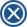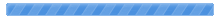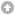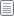Pages: 1Bishops and bitboards (Read 203 times)
 an ordinary chessplayer God Member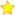OfflineI used to be not bad. Posts: 1637 Location: Columbus, OH (USA) Joined: 01/02/15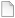Bishops and bitboards 04/18/23 at 22:57:04 I'm constructing some chess lookup tables and came across a matrix property which is interesting enough to share. I can't make any claim to originality: I only know enough about matrices to be dangerous, and since for copyright reasons I generally don't look at other people's code, I only know the prior art in chess programming at a high "name of concept" level. So I may only have rediscovered something which is old hat in one or both of those arenas.The idea of a lookup table is to generate ahead of time all the moves for all the pieces from all the squares. Then in any actual chess position instead of calculating all the legal moves, which is slow, you look them up, which is fast, particularly fast if you use arrays. But it's a lot of pieces and a lot of squares, so of course we want some mathematical formula to help us generate our tables. Another thing that matters in chess is that queens, rooks, and bishops not only move but slide, i.e. they can't have anything between them and their new square. So we need to calculate (later look up) all the in-between moves/squares too. For writing that loop, we need to know how many such squares there are. Ranks and files are all the same size, but diagonals differ, so here we are. We'll use a numbering scheme 0-7 because math with arrays is so much easier that way. Number all the squares from the top left as pairs of rank and file (r,f), e.g. (0,0) (0,1) (0,2) etc. such that the four corners are a8=(0,0) h8=(0,7) a1=(7,0) h1=(7,7). As an aside, we could have started anywhere; we choose this arrangement to simplify FEN processing. We will look at the diagonals NorthWest to SouthEast (NW-SE) and NorthEast to SouthWest (NE-SW). Again, we could have moved in other directions; this particular choice has to do with the sign(+-) of pawn moves.After staring at our (r,f) matrix for a bit, we notice that on any NW-SE diagonal, all the squares have the same difference, rank minus file (r-f), whereas on any NE-SW diagonal, all the squares have the same sum, rank plus file (r+f). Okay, but we care about the _length_ of the diagonals, so how is this helping us? To explore further we create two new tables of (diff,sum,len). Here length (len) is not the total length of the diagonal, instead it's the number of available bishop moves from the (r,f) square towards the South. To simplify the tables, we leave off (r,f), and we pull out diff or sum to the left depending on which one of them is fixed. This latter means the two tables will look a little different for the two directions.NW-SE diagonals arranged in rows as (a1) .. (a8-h1) .. (h8) ```=============== diff | sum,len =====|=========    7 |  7,0 (a1)    6 |  6,1   8,0    5 |  5,2   7,1   9,0    4 |  4,3   6,2   8,1  10,0    3 |  3,4   5,3   7,2   9,1  11,0    2 |  2,5   4,4   6,3   8,2  10,1  12,0    1 |  1,6   3,5   5,4   7,3   9,2  11,1  13,0    0 |  0,7   2,6   4,5   6,4   8,3  10,2  12,1  14,0 (a8-h1)   -1 |  1,6   3,5   5,4   7,3   9,2  11,1  13,0   -2 |  2,5   4,4   6,3   8,2  10,1  12,0   -3 |  3,4   5,3   7,2   9,1  11,0   -4 |  4,3   6,2   8,1  10,0   -5 |  5,2   7,1   9,0   -6 |  6,1   8,0   -7 |  7,0 (h8) =============== ```NE-SW diagonals arranged in rows as (h1) .. (h8-a1) (a8) ```=============== sum | diff,len =====|=========   14 |  0,0 (h1)   13 | -1,1   1,0   12 | -2,2   0,1   2,0   11 | -3,3  -1,2   1,1   3,0   10 | -4,4  -2,3   0,2   2,1   4,0    9 | -5,5  -3,4  -1,3   1,2   3,1   5,0    8 | -6,6  -4,5  -2,4   0,3   2,2   4,1   6,0    7 | -7,7  -5,6  -3,5  -1,4   1,3   3,2   5,1   7,0 (h8-a1)    6 | -6,6  -4,5  -2,4   0,3   2,2   4,1   6,0    5 | -5,5  -3,4  -1,3   1,2   3,1   5,0    4 | -4,4  -2,3   0,2   2,1   4,0    3 | -3,3  -1,2   1,1   3,0    2 | -2,2   0,1   2,0    1 | -1,1   1,0    0 |  0,0 (a8) =============== ```After constructing these tables, just by inspection there is a regular relationship between sum, diff, and len. The first thing jumping out is the rightmost sum (NW-SE) or diff (NE-SW) tracks the total length of the diagonal. Looking a little closer, the interior sum or diff is changing by two while the len (bishop moves) is changing by one. That makes sense because one rank plus one file equals one diagonal (Pythagorus would not be proud of us.)Definitionssum = r+fdiff = r-flen : number of bishop moves from any (r,f) to the right (South)maxsum : NW-SE right-most summaxdiff : NE-SW right-most diffbacklen : number of bishop moves from any (r,f) to the left (North)diagonallength = len + backlen + 1NW-SE formulasmaxsum = 14 - abs(diff)diagonallength = maxsum - 6len = (maxsum - sum)/2backlen = -7 + (maxsum + sum)/2NE-SW formulasmaxdiff = 7 - abs(7-sum)diagonallength = maxdiff + 1len = (maxdiff - diff)/2backlen = (maxdiff + diff)/2There you have it. From any (r,f) it's easy to calculate the number of bishop moves in any direction. Now we can write a loop for the bishop: +/-1 rank +/-1 file for that many moves. I think the relations will hold for any n-by-n board, maybe later I will check what happens on an m-by-n board.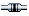IP LoggedPages: 1
 « Board Index ‹ Board